UnboundEd Mathematics Guide

# Operations & Algebraic Thinking: Unbound A Guide to Grade 3 Mathematics Standards

?
What's in a Content Guide and how do I use it?
Get answers to all your Content Guide questions, including what's in each part and how they can be used in your role at your school
View FAQs

3.OA.A | Represent and solve problems involving multiplication and division.

3.OA.B | Understand properties of multiplication and the relationship between multiplication and division.

3.OA.C | Multiply and divide within 100.

3.OA.D | Solve problems involving the four operations, and identify and explain patterns in arithmetic.

Welcome to the UnboundEd Mathematics Guide series! These guides are designed to explain what new, high standards for mathematics say about what students should learn in each grade, and what they mean for curriculum and instruction. This guide, the first for Grade 3, includes three parts. The first part gives a “tour” of the standards in the Operations & Algebraic Thinking domain using freely available online resources that you can use or adapt for your class. The second part shows how multiplication and division relate to other concepts in Grade 3. And the third part explains the progression of learning for multiplication and division with whole numbers in Grades K-5. Throughout all of our guides, we include a large number of sample math problems. We strongly suggest tackling these problems yourself to help best understand the methods and strategies we’re covering, and the potential challenges your students might face.

# Part 1: What do the standards say?

In Grade 3, the Operations & Algebraic Thinking (OA) domain describes some of the important expectations around multiplication and division. The domain is composed of four clusters; each cluster has associated standards and is part of the major work of the grade, as indicated by the green square above.1 The Common Core State Standards for Mathematics (CCSSM) are organized into major, additional and supporting clusters in the Focus by Grade Level documents from Student Achievement Partners. The Common Core State Standards for Mathematics (CCSSM) are organized into major, additional and supporting clusters in the Focus by Grade Level documents from Student Achievement Partners. The Common Core State Standards for Mathematics (CCSSM) are organized into major, additional and supporting clusters in the Focus by Grade Level documents from Student Achievement Partners. A large majority of time should be spent teaching the major work of the grade.

Beginning the year with multiplication and division is a good idea because students are expected to fluently multiply and divide within 100 and know from memory all products of two one-digit numbers by the end of third grade. (3.OA.C.7) The more time we have to develop these fluencies, the better, since they must be grounded in conceptual understanding and fostered through lots of practice (rather than an over-reliance on facts). Conceptual understanding involves a great deal: understanding the meaning and properties of multiplication and division, identifying and explaining patterns, and representing and solving problems involving multiplication and division. Suffice it to say, developing conceptual understanding and using it to support continuous practice will take some time, and starting at the beginning of the year gives us the best chance for that to happen.

It’s important to note that the clusters, and the standards within the clusters, are not necessarily sequenced in the order in which they have to be taught. (Standards are only a set of expectations of what students should know and be able to do by the end of each year; they don’t prescribe an exact sequence or curriculum.) So planning your instruction sequence carefully can ensure your students continue to build on previous understandings. As we go, think about the connections you see between standards, and how you can use these connections to help students build on their previous understandings.

Throughout the guide, we’ll look at examples of tasks and lessons that focus on students’ abilities to make sense of problems and persevere in solving them (MP.1). As students are exposed to varied contexts and problem types, they will need to think carefully to understand each problem and develop an appropriate solution method.

The first cluster in the Operations and Algebraic Thinking domain has four standards. Let’s begin by reading these standards, and then we’ll think through what they mean and how they look in practice.

3.OA.A | Represent and solve problems involving multiplication and division.

 3.OA.A.1 Interpret products of whole numbers, e.g., interpret 5 × 7 as the total number of objects in 5 groups of 7 objects each. For example, describe a context in which a total number of objects can be expressed as 5 × 7. 3.OA.A.2 Interpret whole-number quotients of whole numbers, e.g., interpret 56 ÷ 8 as the number of objects in each share when 56 objects are partitioned equally into 8 shares, or as a number of shares when 56 objects are partitioned into equal shares of 8 objects each. For example, describe a context in which a number of shares or a number of groups can be expressed as 56 ÷ 8. 3.OA.A.3 Use multiplication and division within 100 to solve word problems in situations involving equal groups, arrays and measurement quantities, e.g., by using drawings and equations with a symbol for the unknown number to represent the problem.* 3.OA.A.4 Determine the unknown whole number in a multiplication or division equation relating three whole numbers. For example, determine the unknown number that makes the equation true in each of the equations 8 × ? = 48, 5 = _ ÷ 3, 6 × 6 = ? *See Glossary, Table 2.

## Interpreting products and quotients

Many of us learned multiplication by memorizing our facts and practicing the standard algorithm for a significant amount of time. Though fact fluency and work with algorithms are important, we want students to have the conceptual understanding required for long-term mastery of multiplication and division; if facts and procedures are simply memorized, we run the risk they will be forgotten or misapplied. What’s more, in later grades, students will be able to apply this conceptual understanding of operations with whole numbers to operations with fractions, decimals and eventually all rational numbers and beyond; they won’t have to “relearn” what it means to multiply every time!

#### Grade 3, Module 1, Lesson 3: Problem Set

1. There are 5 flowers in each bunch. How many flowers are in 4 bunches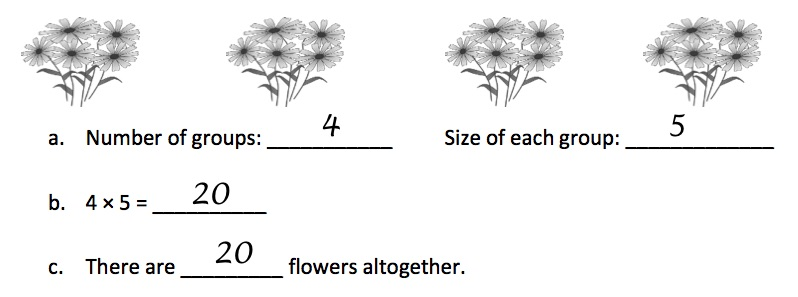Above, the groups are shown as bunches of flowers; there are 5 flowers in each group; and the total, or product, is 20. Instead of representing this problem as repeated addition (i.e., 5 + 5 + 5 + 5 = 20) as students may have done in Grade 2, it is represented using a multiplication equation; in this way, we build on students’ prior learning. In the example below, the situation is represented with an array.

Grade 3, Module 1, Lesson 3: Problem Set

The student uses a level 2 count by multiplication and division strategy to solve the Equal Groups- Unknown Product problem, and explains her answer to her peers. She counts by 5 in order to solve.

#### Grade 3, Module 1, Lesson 4: Application Problem

Application Problem (6 minutes)

The student council holds a meeting in Mr. Chang’s classroom. They arrange the chairs in 3 rows of 5. How many chairs are used in all? Use the RDW process.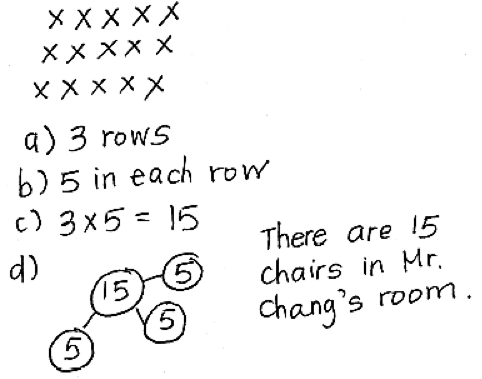Note: This problem reviews relating multiplication to the array model from Lesson 2. Students might choose to solve by drawing an array (Lesson 2) or a number bond (Lesson 3) where each part represents the amount of chairs in each row.

HideShow

Grade 3, Module 1, Lesson 4: Application Problem

The students use two different strategies to solve the Array- Unknown Product problem. One student solves using a level 1 strategy and counts all, and the other solves using a level 2 count by. Both of these strategies are explained by the other students in the class.

In the sample student work, the student relates the multiplication equation (3 x 5 = 15) to the array showing 3 rows of 5. Note that in an array situation, one factor (3) tells the number of rows and the other factor tells the number of columns (5). But this distinction is not important, since a 90-degree rotation causes the rows and columns to switch.3 In fact, this aspect of arrays helps support the commutative property of multiplication, which we will discuss in a bit. Additionally, as described in the note in the application problem, another useful representation for multiplication is a number bond. Students represent the number of equal parts (i.e., rows or groups) with the smaller circles (e.g., there are 3 rows so there are 3 circles) and the size of each part (i.e., the number in each row or group) goes inside the circle (e.g., there are 5 chairs in each row so there is a 5 in each circle). The product is represented by the larger circle. Number bonds, if used by students in prior grades, are a great way to support understanding of multiplication.

In the case of division, students interpret quotients as either the number of shares or the number of objects in each share when a whole number of objects is partitioned equally. (3.OA.A.2) In the “equal groups” task below, students are asked to describe what is meant when the total is divided by the number of fish in each group, and when the total is divided by the number of groups.

#### Fish TanksSuppose there are 4 tanks and 3 fish in each tank. The total number of fish in this situation can be expressed as 4 × 3 = 12.

1. Describe what is meant in this situation by 12 ÷ 3 = 4
2. Describe what is meant in this situation by 12 ÷ 4 = 3

The focus of this task is for students to describe the meaning of each division equation; this supports conceptual understanding of division. Students understand that the quotient can be the number of tanks (4) or the number of fish in each tank (3).

For both multiplication and division, part of interpreting the meaning of products and quotients involves being able to describe a context appropriate for expressing a product or a quotient. (3.OA.A.1, 3.OA.A.2 )Beginning instruction of multiplication and division using real-world contexts establishes meaning for these operations and is parallel with learning addition and subtraction in earlier grades. Understanding and being able to describe the appropriate context for an operation helps students apply operations to solve word problems. In the following example from an assessment, students are asked to determine which context is a multiplication context. The factors in the problem are purposely outside the scope of Grade 3 in order to draw attention to the meaning of the operation.

#### Foundations of Multiplication and Division Mini-Assessment

In which situation is the number of plums equal to 58 × 29?

1. Sam buys 58 plums and puts 29 plums in each of 2 bags.
2. Ron buys 58 bags with 29 plums in each bag.
3. Tim buys 58 plums and gives 29 of the plums away.
4. Dan has 58 plums and buys 29 more plums.

Foundations of Multiplication and Division Mini-Assessment

Students complete an assessment task using a level 3 multiplication and division strategy to solve the Equal Groups-Group Size Unknown problem and explain their answers.

## Multiplication and division problem situations

Many of us have learned that solving word problems involves finding “key words,” — words like “more” and “total” tell us to add, while words like “fewer” and “less” tell us to subtract. But what about a problem like this:

Lucy has six fewer apples than Julie. Lucy has eight apples. How many apples does Julie have?5 Adapted from CCSSM Table 2 Adapted from CCSSM Table 2 Adapted from CCSSM Table 2

The “key word” in this problem, “fewer,” actually hints at the wrong operation; subtracting will not result in the correct answer.

A better way to help students with problem-solving is to help them think situationally about the varied contexts. Using word problems to create meaning for operations helps students to better understand how to apply operations.

### What kinds of multiplication and division problems do students solve in Grade 3?

The table below shows the different multiplication and division problem situations students should master in Grades 3-5, with the first two rows applicable to Grade 3. It will be helpful to spend some time digging into the different problem situations shown in the table, as we will refer to them extensively throughout the rest of this discussion.

Common multiplication and division situations (1)

 UNKNOWN PRODUCT GROUP SIZE UNKNOWN (“HOW MANY IN EACH GROUP?” DIVISION) NUMBER OF GROUPS UNKNOWN (“HOW MANY GROUPS?” DIVISION) 3 x 6 = ? 3 x ? = 18, and 18 ÷ 3 = ? ? x 6 = 18, and 18 ÷ 6 = ? EQUAL GROUPS There are 3 bags with 6 plums in each bag. How many plums are there in all? Measurement example. You need 3 lengths of string, each 6 inches long. How much string will you need altogether? See Video If 18 plums are shared equally into 3 bags, then how many plums will be in each bag? Measurement example. You have 18 inches of string, which you will cut into 3 equal pieces. How long will each piece of string be? See Video If 18 plums are to be packed 6 to a bag, then how many bags are needed? Measurement example. You have 18 inches of string, which you will cut into pieces that are 6 inches long. How many pieces of string will you have? See Video ARRAYS (2), AREA (3) There are 3 rows of apples with 6 apples in each row. How many apples are there? Area example. What is the area of a 3 cm by 6 cm rectangle? See Video If 18 apples are arranged into 3 equal rows, how many apples will be in each row? Area example.A rectangle has area 18 square centimeters. If one side is 3 cm long, how long is a side next to it? See Video If 18 apples are arranged into equal rows of 6 apples, how many rows will there be? Area example. A rectangle has area 18 square centimeters. If one side is 6 cm long, how long is a side next to it? COMPARE A blue hat costs \$6. A red hat costs 3 times as much as the blue hat. How much does the red hat cost? Measurement example. A rubber band is 6 cm long. How long will the rubber band be when it is stretched to be 3 times as long? A red hat costs \$18 and that is 3 times as much as a blue hat costs. How much does a blue hat cost? Measurement example. A rubber band is stretched to be 18 cm long and that is 3 times as long as it was at first. How long was the rubber band at first? A red hat costs \$18 and a blue hat costs \$6. How many times as much does the red hat cost as the blue hat? Measurement example. A rubber band was 6 cm long at first. Now it is stretched to be 18 cm long. How many times as long is the rubber band now as it was at first? GENERAL a x b = ? a x ? = p and p ÷ a = ? ? x b = p, and p ÷ b = ?

(1) The language in the array examples shows the easiest form of array problems. A harder form is to use the terms rows and columns: The apples in the grocery window are in 3 rows and 6 columns. How many apples are in there? Both forms are valuable.

(2) Area involves arrays of squares that have been pushed together so that there are no gaps or overlaps, so array problems include these especially important measurement situations.

(3) The first examples in each cell are examples of discrete things. These are easier for students and should be given before the measurement examples.

Source: CCSSM Table 2

Grade 3, Module 1, Lesson 3: Problem Set

The student uses a level 2 count by multiplication and division strategy to solve the Equal Groups- Unknown Product problem, and explains her answer to her peers. She counts by 5 in order to solve.

Foundations of Multiplication and Division Mini-Assessment

Students complete an assessment task using a level 3 multiplication and division strategy to solve the Equal Groups-Group Size Unknown problem and explain their answers.

Array- Group Size Unknown

The students use a level 3 multiplication and division strategy to decompose one of the factors in this Array- Group Size Unknown Product problem, and explain their answers. They describe what the divisor and dividend both represent to clearly explain their thinking.

Equal Groups- Number of Groups Unknown

The students use two different strategies to solve the Equal Groups- Number of Groups Unknown problem. One student solves using a level 3 strategy to make an easier problem, and the other solves using a level 2 count by. Both of these strategies are explained by other students in the class.

Grade 3, Module 1, Lesson 4: Application Problem

The students use two different strategies to solve the Array- Unknown Product problem. One student solves using a level 1 strategy and counts all, and the other solves using a level 2 count by. Both of these strategies are explained by the other students in the class.

In Grade 3, students use multiplication and division within 100 to solve word problems in situations involving equal groups, arrays and measurement quantities. (3.OA.A.3) These situations are described in the first two rows of Table 2; take a moment to focus on those subtypes, as they are at the heart of our work in Grade 3. Note that the “compare” situations (i.e., multiplicative comparison) described in row three are not introduced until Grade 4. Each subtype in Table 2 shows an example involving discrete objects (e.g., plums) in addition to a measurement example. Another important thing to notice: Problems involving discrete objects are easier than measurement problems, so students should have experience with problems involving discrete objects first.6 Footnote 3 from CCSSM Table 2: The first examples in each cell are examples of discrete things. These are easier for students and should be given before the measurement examples. Footnote 3 from CCSSM Table 2: The first examples in each cell are examples of discrete things. These are easier for students and should be given before the measurement examples. Footnote 3 from CCSSM Table 2: The first examples in each cell are examples of discrete things. These are easier for students and should be given before the measurement examples. So our job in Grade 3 is to provide varied exposure to all of the subtypes in the first two rows, with an initial focus on discrete objects (i.e., plums and apples), followed by measurement and area situations.

As with addition and subtraction problems, multiplication and division problems can have the unknown in any position. Take a moment to check out the three columns in the chart above. In the first column (Unknown Product subtype), the total is unknown; these problems are solved using multiplication. In the second and third columns (Group Size Unknown and Number of Groups Unknown subtypes), one of the factors is unknown. Students might initially solve these kinds of problems using division, but they can also be solved using multiplication if students think of them as unknown factor problems. This happens once students establish the relationship between multiplication and division. (3.OA.B.6) In the course of a unit of instruction, students should be exposed to a variety of problems with unknowns in all positions.

### The language of multiplication problems

Array problems can be written using equal groups language (e.g., There are 3 rows of apples with 6 apples in each row. How many apples are there?) or using row and column language (e.g., The apples in the grocery window are in 3 rows and 6 columns. How many apples are there?). Problems using row and column language are more challenging for students because students may initially have difficulty distinguishing between the number of things in a row and the number of rows (i.e., there are 3 rows and 6 columns which tells us how many are in each row and there are 6 columns and 3 rows which tells us how many are in each column).7 Progressions for the Common Core State Standards in Mathematics (draft): K, Counting and Cardinality; K–5, Operations and Algebraic Thinking, p. 24. Progressions for the Common Core State Standards in Mathematics (draft): K, Counting and Cardinality; K–5, Operations and Algebraic Thinking, p. 24. Progressions for the Common Core State Standards in Mathematics (draft): K, Counting and Cardinality; K–5, Operations and Algebraic Thinking, p. 24. Using equal groups language in array problems serves as a good intermediary for students when bridging their understanding between the equal groups problems and array problems that use row and column language.

An important note about conventions of writing multiplication equations: In the United States, we typically write the number of groups first for equal groups problems (e.g., 3 x 6 = ? means there are 3 groups of 6). While this might be typical, it is not universal. In fact, in many other countries the reverse is true (e.g., 3 x 6 = ? means there are 6 groups of 3). More important than the convention of writing groups first or second is understanding that the factors have meaning and students should understand their meaning. Additionally, this is also true of array problems: writing rows first is not required. As we’ve discussed, rotating the orientation by 90 degrees interchanges the rows and columns. Understanding the language of rows and columns is more important.8 Ibid. p. 25. Ibid. p. 25. Ibid. p. 25.

### Representations of multiplication and division word problems in Grade 3

So what does it look like when students solve multiplication and division word problems in Grade 3? Students represent multiplication and division word problems using equations with symbols for the unknown. (3.OA.A.3 )Students also use drawings (e.g., equal groups, arrays, area models, number bonds, and tape diagrams) to represent problem situations. The example below uses the context of money (work with whole dollar amounts begins in Grade 2 (2.MD.C.8)).

#### Gifts From Grandma, Variation 1

1. Juanita spent \$9 on each of her 6 grandchildren at the fair. How much money did she spend?
2. Nita bought some games for her grandchildren for \$8 each. If she spent a total of \$48, how many games did Nita buy?
3. Helen spent an equal amount of money on each of her 7 grandchildren at the fair. If she spent a total of \$42, how much did each grandchild get?

Solutions

Solution: Tape diagram

This task needs a tape diagram solution.

Solution: Writing multiplication equations for division problems

1. Sandra spent 6 groups of \$9, which is 6 × 9 = 54 dollars all together.
2. Since the number of games represent the number of groups, but we don’t know how many games she bought, this is a "How many groups?" division problem. We can represent it as

? × 8 = 48

or

48 ÷ 8 = ?

So Nita must have bought 6 games.

1. Here we know how many grandchildren there are (so we know the number of groups), but we don’t know how much money each one gets (the number of dollars in each group). So this is a "How many in each group?" division problem. We can represent it as

7 × ? = 42

or

42 ÷ 7 = ?

So Helen must have given each grandchild \$6.

HideShow

The student solves the Equal Groups- Group Size Unknown problem with a level 3 multiplication and division strategy to make an easier problem. He decomposes the dividend to solve.

The task shows three equal groups problems in which the unknown is in a different position. The first problem is a multiplication problem in which the product is unknown (Unknown Product). The next two problems show two related division/unknown factor problems: "How many groups?" (Number of Groups Unknown) and "How many in each group?" (Group Size Unknown). The sample solutions shown in the task use a question mark to represent the unknown. For example, in problem b, students might represent the problem using the equation ? x 8 = 48, where the unknown is a factor. Or students might represent the problem using the equation 48 ÷ 8 = ?, where the unknown is the quotient. It’s important that students are given opportunities to see how different equations can represent the same situation.

We’ve talked so far about interpreting products and quotients and about applying multiplication and division to solve problems. As students become more secure in their understanding of multiplication and division, they will be able to determine the unknown in a multiplication or division equation more abstractly (equations that are not tied to a particular context). (3.OA.A.4) The task below is designed to draw out student misconceptions about unknowns in division equations. The problem does not have a context; students have to make sense of the relationship between the numbers and reason about their answer.

#### Finding the Unknown in a Division Equation

Tehya and Kenneth are trying to figure out which number could be placed in the box to make this equation true.

Tehya insists that 12 is the only number that will make this equation true.

Kenneth insists that 3 is the only number that will make this equation true.

2 = ⎕6

Who is right? Why? Draw a picture to support your idea.

Note the way the task asks students to draw a picture and the “unconventional” arrangement of the equal sign and unknown; both of these characteristics serve to build flexible thinking and conceptual understanding.

## Properties of multiplication and the relationship between multiplication and division

The second cluster in the Operations and Algebraic Thinking domain describes the properties of multiplication and the relationship between multiplication and division. Let’s read the standards associated with this cluster, and then we’ll think through what they mean and how they look in practice.

3.OA.B | Understand properties of multiplication and the relationship between multiplication and division.

 3.OA.B.5 Apply properties of operations as strategies to multiply and divide.* Examples: If 6 × 4 = 24 is known, then 4 × 6 = 24 is also known. (Commutative property of multiplication.) 3 × 5 × 2 can be found by 3 × 5 = 15, then 15 × 2 = 30, or by 5 × 2 = 10, then 3 × 10 = 30. (Associative property of multiplication.) Knowing that 8 × 5 = 40 and 8 × 2 = 16, one can find 8 × 7 as 8 × (5 + 2) = (8 × 5) + (8 × 2) = 40 + 16 = 56. (Distributive property.) 3.OA.B.6 Understand division as an unknown-factor problem. For example, find 32 ÷ 8 by finding the number that makes 32 when multiplied by 8. *Students need not use formal terms for these properties.

## The relationship between multiplication and division

Quick: What’s 100 divided by 5? Did you compute a division problem in your head? Or did you recognize 20 as the number, when multiplied by 5, gives 100? Mathematically fluent adults can quickly divide by understanding division as an unknown factor problem; (3.OA.B.6) we want the same for our students. Developing this understanding allows students to understand the relationship between multiplication and division and to use this relationship to solve problems. Establishing this connection should happen very early when teaching division and can even be a good starting point in the transition from multiplication to division. The following lesson shows one way in which students connect the quotient to an unknown factor. In the two lessons prior to this one, students were introduced to division in terms of finding the number of groups or the number of objects in the groups (i.e., the factors).

#### Grade 3, Module 1, Lesson 6: Concept Development

Problem 2: Use an array to relate the unknown factor in multiplication to the quotient in division.

T: Draw an array that shows the equation 15 ÷ 3 = 5 where the quotient—that means the answer—represents the size of the groups.

S: (Draw array below.)T: Now, write both a division and a multiplication equation for the array.

S: (Write 15 ÷ 3 = 5, 3 × 5 = 15.)

T: Where do you find the quotient in our multiplication equation?

S: It’s the second number. → It’s the size of the groups. → It’s a factor.

T: Circle the size of the groups in both problems.

S: (Circle the 5 in both problems.)

Repeat the process with the following suggested examples. Alternate between having the quotient represent the size of the groups and the number of groups.

• 4 rows of 2
• 7 rows of 3

T: Use our equations to explain to your partner how the factors in a multiplication problem can help you find the quotient in division.

HideShow

In the lesson, an array is used as a visual support for students in understanding division as an unknown factor problem. The key question that the teacher asks is: “Where do you find the quotient in our multiplication equation?” The answers that the students give (“It’s the second number,” “It’s the size of the groups,” “It’s a factor”) show that they are connecting finding a quotient to finding a missing factor.

In the next example, students are asked to recognize and explain the relationship between multiplication and division: The quotient is the same as an unknown factor.

#### Foundations of Multiplication and Division Mini-Assessment

1. Amy and Bonnie are putting an equal number of stickers into 5 sticker books. They have 100 stickers total.

Amy wrote the following division equation to find the number of stickers to put in each sticker book:

100 ÷ 5 = _____

Bonnie wrote the following multiplication equation to find the number of stickers to put in each sticker book:

5 × _____ = 100

Who is correct? (Circle one answer. )

 Neither person Amy Bonnie Both people

HideShow

## Levels of multiplication and division strategies

Over the course of Grade 3, students will progress across three levels of multiplication and division strategies, which align closely with the levels for addition and subtraction.9 The Level 1 method for addition and subtraction is counting all, Level 2 is counting on, and Level 3 is making an easier problem by decomposing and composing numbers. See a discussion on these levels in the Grade 1 Guide. The Level 1 method for addition and subtraction is counting all, Level 2 is counting on, and Level 3 is making an easier problem by decomposing and composing numbers. See a discussion on these levels in the Grade 1 Guide. The Level 1 method for addition and subtraction is counting all, Level 2 is counting on, and Level 3 is making an easier problem by decomposing and composing numbers. See a discussion on these levels in the Grade 1 Guide. The progression begins with “counting all” and ends with applying properties (associative and distributive), (3.OA.B.5) which are part of the highest level.10 Progressions for the Common Core State Standards in Mathematics (draft): K, Counting and Cardinality; K–5, Operations and Algebraic Thinking, pp. 25-26. Progressions for the Common Core State Standards in Mathematics (draft): K, Counting and Cardinality; K–5, Operations and Algebraic Thinking, pp. 25-26. Progressions for the Common Core State Standards in Mathematics (draft): K, Counting and Cardinality; K–5, Operations and Algebraic Thinking, pp. 25-26. Understanding these levels will help us ensure we’re moving our students along. Let’s take a closer look.

The Level 1 method is making (either using pictures or manipulatives) and counting all of the quantities in a multiplication or division situation. Students engage in Level 1 representations and computations beginning in Grade 2 when students explore even numbers and repeated addition with objects arranged into rectangular arrays. (2.OA.C.3, 2.OA.C.4) Using the example from Table 2 in the CCSSM (There are 3 bags with 6 plums in each bag. How many plums are there in all?), a student might draw 3 bags and 6 plums in each bag, and then count all of the plums to find the product.

Grade 3, Module 1, Lesson 4: Application Problem

The students use two different strategies to solve the Array- Unknown Product problem. One student solves using a level 1 strategy and counts all, and the other solves using a level 2 count by. Both of these strategies are explained by the other students in the class.

The Level 2 method is repeated counting on by a given number, also called “count-bys” or skip-counting. In the example below, students would count by 2’s four times, keeping track of the number of 2’s they count. In the case of division, 8 ÷ 2, students count by 2’s until they reach 8, keeping track of the number of 2’s they count. In addition to lots of practice with skip-counting another way to help students move from Level 1 to Level 2 is to use small arrays and write the running total for each row, as demonstrated in the example below.

Grade 3, Module 1, Lesson 3: Problem Set

The student uses a level 2 count by multiplication and division strategy to solve the Equal Groups- Unknown Product problem, and explains her answer to her peers. She counts by 5 in order to solve.

#### Grade 3, Module 1, Lesson 7: Concept Development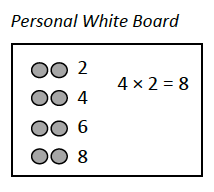As students become more secure with skip-counting, they can move away from using drawings that show all of the quantities and toward using diagrams (e.g., number bonds, tape diagrams, area models). Diagrams represent situations by showing the relationship of numbers.11 Progressions for the Common Core State Standards in Mathematics (draft): K, Counting and Cardinality; K–5, Operations and Algebraic Thinking, p. 25. Progressions for the Common Core State Standards in Mathematics (draft): K, Counting and Cardinality; K–5, Operations and Algebraic Thinking, p. 25. Progressions for the Common Core State Standards in Mathematics (draft): K, Counting and Cardinality; K–5, Operations and Algebraic Thinking, p. 25. In the examples below, students use a tape diagram and a number bond to show division.

#### Grade 3, Module 1, Lesson 17: Problem Set

1. The baker packs 36 bran muffins in boxes of 4. Draw and label a tape diagram to find the number of boxes he packs.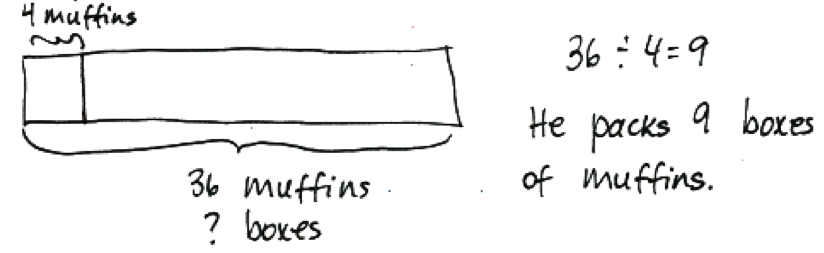1. The waitress arranges 32 glasses into 4 equal rows. How many glasses are in each row?HideShow

In problem 2, students use division to solve the problem and use the number 4 in the tape diagram rather than drawing out 4 muffins. In problem 3, students solve the problem as an unknown factor problem and use skip-counting to determine the unknown factor. Then they represent the problem situation using a division equation, identifying the number of glasses in each row as the quotient.

Level 3 methods for multiplying and dividing involve making an easier problem by using the associative, distributive, or commutative properties, in addition to decomposing numbers. Decomposing and composing numbers is a skill that students have been developing since Kindergarten, with respect to addition and subtraction. In Grade 3, students extend this understanding to multiplication and division.

Work with properties is important and worthy of some more of our time. Let’s take a closer look.

Foundations of Multiplication and Division Mini-Assessment

Students complete an assessment task using a level 3 multiplication and division strategy to solve the Equal Groups-Group Size Unknown problem and explain their answers.

Array- Group Size Unknown

The students use a level 3 multiplication and division strategy to decompose one of the factors in this Array- Group Size Unknown Product problem, and explain their answers. They describe what the divisor and dividend both represent to clearly explain their thinking.

## Properties of operations12 Table 3 of the CCSSM describes the properties of operations Table 3 of the CCSSM describes the properties of operations Table 3 of the CCSSM describes the properties of operations

Its important to note that this is not the first time students have engaged with properties of operations; underlying students work with addition and subtraction in Grades 1-2 is an understanding of the properties of addition. (1.OA.C.6) You will want to ensure that students employ the commutative and associative properties of multiplication and the distributive property toward establishing fluency with single-digit facts. Note that the Standards dont require that students know the names of these properties at this level, as it distracts from conceptual understanding of these properties. The focus is always on applying these properties as strategies to multiply and divide. (3.OA.B.5 )Students don’t learn properties just for some intrinsic value; rather they are always framed as something helpful for solving problems.

### The associative property of multiplication

The associative property of multiplication tells us that we can choose any grouping of factors and get the same product; for example, (2 x 3) x 4 and 2 x (3 x 4) both yield 24. How does this help with multiplication? For two numbers being multiplied, students can use the associative property, after decomposing one of the numbers multiplicatively into its factors, to make an easier multiplication fact. For example, when multiplying 16 x 3, a student might decompose 16 into 8 x 2 and use the associative property to make an easier fact of 8 x 6:

16 x 3 = (8 x 2) x 3 = 8 x (2 x 3) = 8 x 6 = 48

The understanding behind the associative property of multiplication should be developed conceptually, using concrete and/or pictorial representations. An example of a lesson that does this can be found here: Grade 3, Module 3, Lesson 9.

The Class Trip

The students solve the two- step word problem with level 3 multiplication and division strategies to make an easer problem. Students choose to solve using the associative and distributive properties.

### The distributive property

The distributive property relates multiplication and addition; for example, both 2 x (3 + 4) and 2 x 3 + 2 x 4 result in 14. Similar to what we just looked at with the associative property, students can also make an easier multiplication problem by decomposing one of the factors into its addends (additive decomposition) and then using the distributive property. Again, we can build conceptual understanding through the use of visuals. The following example comes from an EngageNY lesson on the distributive property. In the concept development part of the lesson, students use the 5 + n pattern to decompose a factor into its addends (i.e., the numbers 6, 7, 8, and 9 can be thought of as 5 + 1, 5 + 2, 5 + 3, and 5 + 4 respectively). Then they multiply the easier facts and find the sum. In the homework problem below, the 8 is decomposed into 5 + 3 because knowing facts of 5 (i.e., 5 x 4) and 3 (i.e., 3 x 4) is easier for students.

#### Grade 3, Module 1, Lesson 16: Homework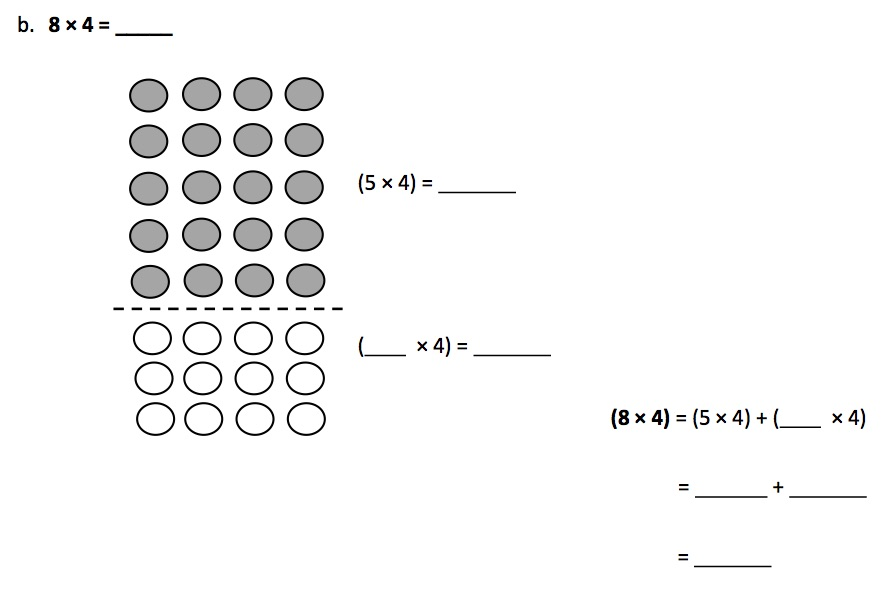The distributive property is introduced and used when multiplying single-digit numbers to help students strategically progress toward fluency with the more challenging facts. For example, instead of rote memorization of 8 x 4, students can use the distributive property to multiply easier facts that they may be more fluent with, like (4 x 4) + (4 x 4) or (2 x 4) + (6 x 4). Students continue to use these properties as they move on to multiplying single-digit numbers by 2-digit numbers (with products within 100 in Grade 3). And believe it or not, In later grades, we’ll see that the distributive property is at the root of understanding the standard algorithm for multiplication.

The Class Trip

The students solve the two- step word problem with level 3 multiplication and division strategies to make an easer problem. Students choose to solve using the associative and distributive properties.

### The commutative property of multiplication

The commutative property lets us know we can change the order of the factors in a multiplication expression; for example, 2 x 3 and 3 x 2 both result in 6. The commutative property will help students access a wider range of facts; for example, if students know 9 x 6 then they also know 6 x 9. To introduce the commutative property, we can again turn to our trusty arrays to build conceptual understanding. The following lesson from EngageNY introduces students to the commutative property of multiplication by using an array and then rotating the array 90 degrees. As observed, the product is the same, however, the position of the factors changed.

#### Grade 3, Module 1, Lesson 7: Concept Development

Concept Development (32 minutes)

Materials: (S) Personal white board

Problem 1: Rotate arrays 90 degrees.

T: Position your board so that the long side is horizontal. Draw an array that shows 4 rows of 2.

S: (Draw the array, as shown here)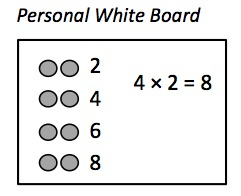T: Write a skip-count by twos to find the total. Then write a multiplication sentence where the first factor represents the number of rows.

S: (Write 2, 4, 6, 8 and 4 × 2 = 8 as shown)

T: Rotate your board 90 degrees so that the long side is vertical.

S: (Rotate, as shown here.)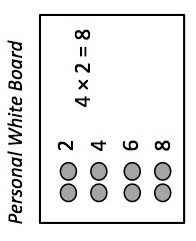T: What happened to the array?

S: It has 2 rows of 4. → It has 4 groups of 2, but they’re up and down instead of in rows.

T: Now the twos are columns, vertical groups in an array.

T: I’ll rotate my board. You tell me if the twos are columns or rows.

T: (Show the twos as rows.)

S: Rows!

T: (Rotate your board and show the twos as columns.)

S: Columns!

T: Skip-count the rows by four!

S: (Point to the rows as students count.) 4, 8.

T: Add that skip-count to your board. (Allow time.) What multiplication sentence can represent this array?

S: 2 × 4 = 8.

T: (Write 4 × 2 = 8 and 2 × 4 = 8 on the board with their corresponding arrays drawn as shown.) What do you notice about the multiplication sentences?S: The 4 and the 2 switched places.

T: What do the 4 and 2 represent in each? Talk to your partner.

S: In A, the 4 represents the number of rows, but in B, it represents the size of the row. → The twos are rows in A but columns in B.

T: Did the meaning of the 8 change?

S: No.

T: So factors can switch places and trade meanings, but the total stays the same. We call that the commutative property. Talk to your partner about why the total stays the same.

S: (Discuss.)

Continue with 2 × 5 and 3 × 4 arrays.

HideShow

The commutative property helps students recognize equivalence of multiplication facts. In the lesson above, 4 rows of 2 and 2 rows of 4 have the same product. Thus, 4 x 2 = 2 x 4. Students can apply the commutative property as a strategy for knowing more multiplication facts. If students know 4 x 2, then they also know 2 x 4. This is especially useful when students are working toward fluency with the more challenging single-digit numbers.

## Fluency with multiplication and division

The third cluster in the Operations & Algebraic Thinking domain describes the expected fluencies for multiplication and division in Grade 3. Let’s read the standard associated with this cluster, and then we’ll think through what it means and how it looks in practice.

3.OA.C | Multiply and divide within 100.

 3.OA.C.7 Fluently multiply and divide within 100, using strategies such as the relationship between multiplication and division (e.g., knowing that 8 × 5 = 40, one knows 40 ÷ 5 = 8) or properties of operations. By the end of Grade 3, know from memory all products of two one-digit numbers.

The first part of this standard describes that students should be able to fluently multiply and divide within 100. By “fluently,” it is meant that students should be able to multiply and divide numbers within 100, with relative speed and accuracy, by using their understanding of the relationship between multiplication and division, by applying properties of operations, and by using their understanding patterns in multiplication (see 3.OA.D.9 below).13 Progressions for the Common Core State Standards in Mathematics (draft): K, Counting and Cardinality; K–5, Operations and Algebraic Thinking, p. 27. Progressions for the Common Core State Standards in Mathematics (draft): K, Counting and Cardinality; K–5, Operations and Algebraic Thinking, p. 27. Progressions for the Common Core State Standards in Mathematics (draft): K, Counting and Cardinality; K–5, Operations and Algebraic Thinking, p. 27. This includes, for example, multiplying 17 x 5, possibly using the distributive property (e.g., 17 x 5 = (10 x 5) + (7 x 5) = 50 + 35 = 85). In Grade 3, multiplying within 100 is based on fluency with strategies rather than algorithms. Students should be able to engage strategies to perform these operations with accuracy and relative speed.

The second part of the standard states that students should know from memory the products of two one-digit numbers by the end of the year. As mentioned, knowing these facts from memory is rooted in conceptual understanding and developed through sustained practice. Students should have daily opportunities to practice multiplication and division of facts they have learned. This might include daily skip-counting activities with opportunities to relate skip-counting to multiplication and division equations or other activities that promote fluency. The following fluency mini-assessment, developed by Student Achievement Partners, assesses fluency with two one-digit numbers. The unknowns are placed in all positions to emphasize the relationship between factors and quotients.

#### Multiplication and Division Within 100 Mini-Assessment

Name: ______________________ Date: ________

 9 × 2 = ___ ___ × 7 = 56 24 ÷ 6 = ___ 5 × 8 = ___ 7 × 6 = ___ 27 ÷ 3 = ___ 35 ÷ 5 = ___ 64 ÷ 8 = ___ 9 × ___ = 36 ___ × 7 = 21 2 × 4 = ___ 45 ÷ 5 = ___ 3 × 3 = ___ 14 ÷ 7 = ___ 36 ÷ 6 = ___ 8 × ___ = 32 7 × 7 = ___ 5 × ___ = 25 ___ × 2 = 12 28 ÷ 4 = ___

## Using all four operations and arithmetic patterns

The fourth cluster in the Operations & Algebraic Thinking domain describes problem-solving using all four operations and examining arithmetic patterns. Let’s read the standards associated with this cluster, and then we’ll think through what they mean and how they look in practice.

3.OA.D | Solve problems involving the four operations, and identify and explain patterns in arithmetic.

 3.OA.D.8 Solve two-step word problems using the four operations. Represent these problems using equations with a letter standing for the unknown quantity. Assess the reasonableness of answers using mental computation and estimation strategies including rounding.* 3.OA.D.9 Identify arithmetic patterns (including patterns in the addition table or multiplication table), and explain them using properties of operations. For example, observe that 4 times a number is always even, and explain why 4 times a number can be decomposed into two equal addends. *This standard is limited to problems posed with whole numbers and having whole-number answers; students should know how to perform operations in conventional order when there are no parentheses to specify a particular order (Order of Operations).

## Arithmetic patterns

Students should have opportunities to identify and explain arithmetic patterns to support developing meaning of operations. (3.OA.D.9 )There are many patterns to explore, and it’s important that students identify and explain patterns with both addition and multiplication. As mentioned previously, students can use addition patterns like 5 + n to support multiplication using the distributive property.

You’ve probably at some point noticed a pattern in the multiples of 9; indeed, there are many! For example, you might have noticed that the multiples of 9 keep moving “away” from the next ten (i.e., 9 is “1 away” from 10, 18 is “2 away” from 20, 27 is “3 away” from 30, etc.). Believe it or not, students in Grade 3 can not only identify but explain patterns when multiplying by 9 (i.e., 9 = 10 – 1); this work ties together multiplication and properties in service of explaining and identifying patterns. The example below uses the distributive property after decomposing 9 into 10 – 1.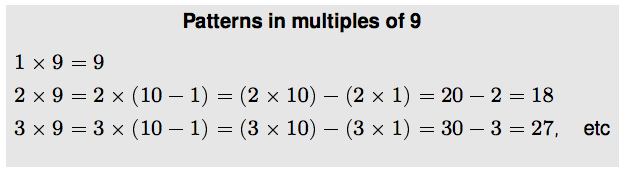Students can explore and describe the pattern that emerges: When taking any number of groups of size 9, the result is the number of groups times ten minus the number of groups. Students can also draw the conclusion that the sum of the digits in the product is equal to 9 (when multiplying 9 by any number 1-9).14 To see a detailed exploration of patterns with multiplying by 9, review lessons 12-14 in Module 3 of the EngageNY curriculum. To see a detailed exploration of patterns with multiplying by 9, review lessons 12-14 in Module 3 of the EngageNY curriculum. To see a detailed exploration of patterns with multiplying by 9, review lessons 12-14 in Module 3 of the EngageNY curriculum. It is also important to distinguish the patterns for multiplying by 1 and by 0 from the patterns of adding 1 and adding 0, because students often confuse these patterns.15 Progressions for the Common Core State Standards in Mathematics (draft): K, Counting and Cardinality; K–5, Operations and Algebraic Thinking, p. 26. Progressions for the Common Core State Standards in Mathematics (draft): K, Counting and Cardinality; K–5, Operations and Algebraic Thinking, p. 26. Progressions for the Common Core State Standards in Mathematics (draft): K, Counting and Cardinality; K–5, Operations and Algebraic Thinking, p. 26.

## Using the four operations to solve problems

Students should also have many opportunities to solve two-step word problems involving all four operations. (3.OA.D.8 )Students began work with two-step problems in Grade 2, but those were limited to addition and subtraction contexts. In Grade 3, students work with two-step problems involving any combination of the four operations. In addition, formal use of algebraic language begins in Grade 3 with use of a letter for the unknown. While students have been using a symbol for the unknown since first grade, specifically using a letter for the unknown begins in Grade 3. Consistent use of letters to represent unknowns in Grade 3 is vital for all future work with algebra.

Two-step problems may require one representation and solution or more difficult problems may require two representations and solutions. The task below illustrates a multiplication and subtraction problem in which the solution is shown in two steps and an equation using a letter for the unknown is used to represent the problem.

#### The Class Trip

Mrs. Moore’s third grade class wants to go on a field trip to the science museum.

• The cost of the trip is \$245.
• The class can earn money by running the school store for 6 weeks.
• The students can earn \$15 each week if they run the store.

1. How much more money does the third grade class still need to earn to pay for their trip?

1. Write an equation to represent this situation.

Solution

1. We can start by finding out how much money the students can make at the store: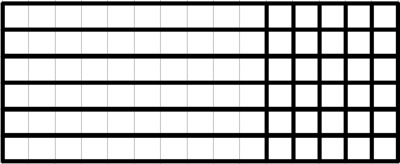6 × 15 = 6 × 10 + 6 × 5 = 60 + 30 = 90

Since

245 − 90 = 155

the students still need \$155 dollars for the field trip.

1. We can let n stand for the amount of money they still need. We know that the amount they can make at the store is 6×15 and the amount they need to raise is 245, so one equation is

245 − 6 × 15 = n

Another possible equation is

6 × 15 + n = 245

The Class Trip

The students solve the two- step word problem with level 3 multiplication and division strategies to make an easer problem. Students choose to solve using the associative and distributive properties.

As part of solving two-step problems, students may use both diagrams and equations. In the example above, the solution shows the use of an area model to multiply 6 x 15 and shows an equation to subtract 245 – 90. Then students reason about the solution steps (i.e., multiplication and subtraction) in order to represent the entire problem with one equation.

Students have to understand two conventions about the order of operations when reading and writing expressions that have more than one operation. Understanding these conventions allows students to represent two-step and multi-step situations with one equation.

• First, students have to understand that they should compute the operation inside the parentheses before computing the operation outside of the parentheses. This is important to understand when students learn about the distributive and associative properties.
• The second convention is that when multiplication or division is next to addition and subtraction, students can imagine parentheses around the multiplication or division (i.e., this operation is done first).

The second part of 3.OA.D.8 deals with assessing the reasonableness of answers using mental computation and estimation strategies, including rounding. Additionally, the ability to determine which operations are required for a particular context is critical to assessing reasonableness. Students might be asked to consider the reasonableness of a solution for part of a problem or to the problem as a whole. Also, they might also be asked to think about what a reasonable answer might be before engaging in the actual computation. For example, using the task above (The Class Trip), a teacher might get students thinking about reasonableness by asking the following question.

• Before solving the problem, think about the following: Would you expect the amount of money the class needs to earn to be, less than \$100, between \$100 and \$200, between \$200 and \$245, or more than \$245? Explain your reasoning.

This question encourages students to think about what is being asked in the problem and what should happen if you subtract a positive number from a larger number. They are also being asked to consider the size of the factors and what would be a reasonable product to subtract from 245. Additionally, they are being asked to consider what would be a reasonable-sized difference when subtracting some number greater than 60 (because 6 x 10 = 60) and less than 120 (because 6 x 20 = 120) from 245.

# Part 2: How do Operations & Algebraic Thinking relate to other parts of grade 3?

## Multiplying with multiples of 10

It is expected that students multiply one-digit whole numbers by multiples of 10 between 10 and 90. (3.NBT.A.3) This is in addition to being able to multiply and divide within 100. Students can use place value strategies to multiply by multiples of 10. The EngageNY lesson below shows how to use the place value chart to illustrate the concept of multiplying by a multiple of 10.

#### Grade 3, Module 3, Lesson 19: Concept Development

Problem 2: Multiply by multiples of 10 using a place value chart.

T: (Project or draw the place value chart shown here.)

Use the chart to write an equation in both unit form and standard form.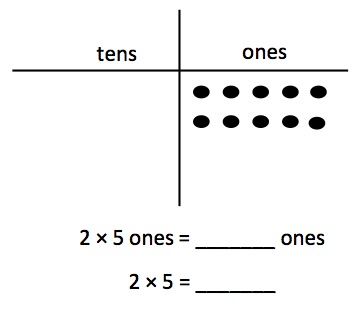S: (Write 2 × 5 ones = 10 ones and 2 × 5 = 10.)

T: How many ones do I have in total?

S: 10 ones.

T: (Project or draw the place value chart shown here.) Compare the two charts. What do you notice about the number of dots?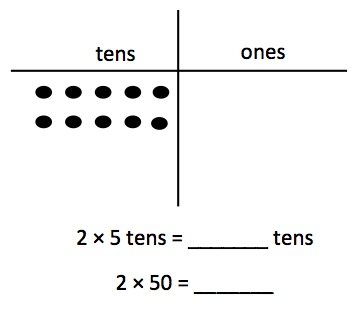S: The number of dots is exactly the same in both charts. → The only thing that changes is where they are placed. The dots moved over to the tens place.

T: Because we still have a total of ten dots, what change do you think we will make in our equations?

S: The units will change from ones to tens.

S: (Write equations.)

T: Say the full equation in standard form.

S: 2 times 50 equals 100.

HideShow

Similarly, using the associative property to multiply is an important strategy. Using the example from the standard (9 x 80), the 80 is decomposed into factors (8 x 10), and using the associative property the new factors become 72 x 10, which is 72 tens, or 720.

9 x 80 = 9 x (8 x 10) = (9 x 8) x 10 = 72 x 10 = 720

## Rounding

Students should learn rounding to the nearest 10 and 100 using place value understanding. (3.NBT.A.1 )This is in contrast to memorizing rules about digits in the tens and ones place. Many of us learned to round by memorizing rules or even rhymes (“5 or more, Raise the score, 4 or less, Give it a rest!”) However, memorizing rules without developing understanding is not enduring for many students. Using a number line to teach rounding helps students visualize how numbers are closer to a particular ten or hundred; it is strategy based on students’ existing number sense. While we certainly wouldn’t expect students to draw a number line every time they round for the duration of their mathematical lives, the visual representation helps to form a solid conceptual basis for rounding. The task below, introduces rounding to the nearest 10 and 100 using a number line.

#### Rounding to the Nearest Ten and Hundred

Plot 8, 32, and 79 on the number line.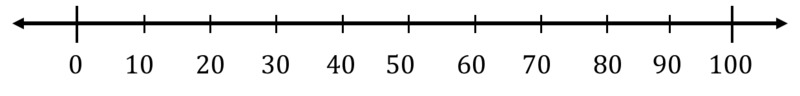1. Round each number to the nearest 10. How can you see this on the number line?
2. Round each number to the nearest 100. How can you see this on the number line?

HideShow

Rounding helps students with estimation strategies and determining reasonableness. In the task, The Class Trip, shown in Part 1, students might determine that an answer of 155 is reasonable, because 90 rounded to the nearest hundred is 100 and 245 – 100 = 145, which is close to 155.

## Area

In Grade 3, students are expected to relate area to the operations of multiplication and addition. (3.MD.C.7) Initially, students understand that area is an attribute of plane figures and that unit squares can be used to measure area in terms of square units. (3.MD.C.5 )They relate rectangular areas to rectangular arrays by tiling with unit squares; building on prior learning, the tiling looks like an array. Then students show that the area of a rectangle can be determined by multiplying the side lengths, which correspond to the number of rows and columns in the array. Put another way, the number of square units in one row multiplied by the number of square units in one column yields the total number of square units (the area of the rectangle). The example below illustrates this point, and shows one way to transition students from thinking in terms of discrete objects in an array to the concept of area.

#### Grade 3, Module 4, Lesson 7: Homework

1. Find the area of each rectangular array. Label the side lengths of the matching area model, and write a multiplication equation for each area model.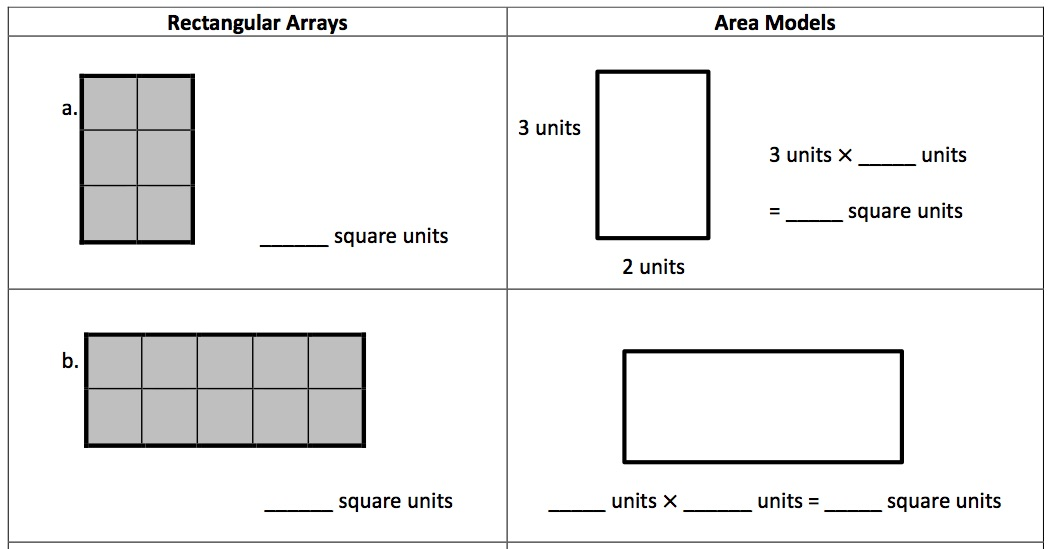The focus on area contexts reinforces understanding of multiplication and division, including the use of properties of operations. For example, students use area models to understand and represent the distributive property (3.MD.C.7.C) as shown in the example below:

#### Grade 3, Module 4, Lesson 10: Problem Set

1. Label the side lengths of the shaded and unshaded rectangles when needed. Then, find the total area of the large rectangle by adding the areas of the two smaller rectangles.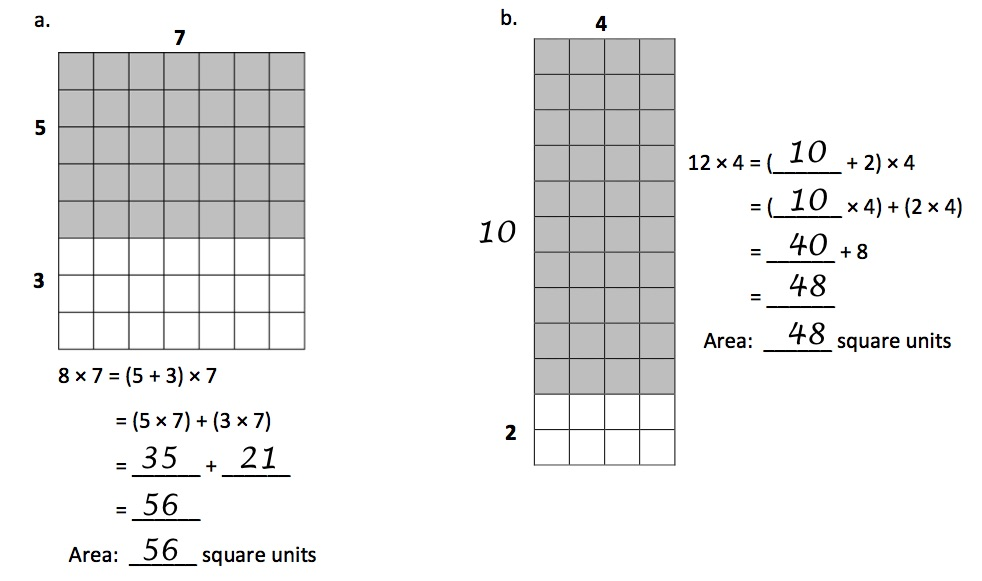HideShow

Students find the area of the shaded and unshaded parts by multiplying the sides lengths and then find the total area of the rectangle by adding the area of each part. In this way, students also learn that areas are additive. (3.MD.C.7.D)

# Part 3: Where do Operations & Algebraic Thinking come from, and where are they going?

Podcast clip: Importance of Coherence with Andrew Chen and Peter Coe (start 9:34, end 26:19)

## Where do Operations & Algebraic Thinking in Grade 3 come from?

Students focus intently on the development of the ideas of addition and subtraction in Kindergarten through Grade 2. This study is characterized by a balance of deep conceptual understanding, development of fluency with these operations, and gradual exposure to more and varied problem-solving contexts.

These prerequisites are obviously important for problem-solving with all four operations in Grade 3. (3.OA.D.8) But what do the foundations of addition and subtraction have to do with learning to multiply and divide? Thorough understanding of addition and subtraction in K-2 is critical for developing understanding of multiplication and division for a few reasons:

• Students draw on their understanding of properties, the relationship between addition and subtraction, and decomposition and composition of numbers to multiply and divide.21 Progressions for the Common Core State Standards in Mathematics (draft): K, Counting and Cardinality; K–5, Operations and Algebraic Thinking, p. 18-19. Progressions for the Common Core State Standards in Mathematics (draft): K, Counting and Cardinality; K–5, Operations and Algebraic Thinking, p. 18-19. Progressions for the Common Core State Standards in Mathematics (draft): K, Counting and Cardinality; K–5, Operations and Algebraic Thinking, p. 18-19. In fact, there are many parallels between the study of addition and subtraction in K-2 and the study of multiplication and division in Grades 3-5.
• Students will again use the commutative and associative properties as strategies, this time for multiplication and division; decomposition will also play a critical role as students understand the distributive property (e.g., being able to see an expression like 8 x 3 as (4 + 4) x 3 which is equivalent to 4 x 3 + 4 x 3).
• Exploring and being able to apply the relationship between multiplication and division is similar to the relationship between addition and subtraction.

• Additionally, facility with using addition and subtraction to solve word problems helps students to better distinguish multiplication and division from addition and subtraction.22 Progressions for the Common Core State Standards in Mathematics (draft): K, Counting and Cardinality; K–5, Operations and Algebraic Thinking, p. 19. Progressions for the Common Core State Standards in Mathematics (draft): K, Counting and Cardinality; K–5, Operations and Algebraic Thinking, p. 19. Progressions for the Common Core State Standards in Mathematics (draft): K, Counting and Cardinality; K–5, Operations and Algebraic Thinking, p. 19. Put another way, students can better understand contexts for which multiplication or division is the appropriate operation if they are secure in their understanding of contexts that require addition or subtraction. Mastery of the problem types described in Table 1 of the Common Core State Standards for Mathematics is an important part of developing this security.23 CCSSM Table 1 CCSSM Table 1 CCSSM Table 1 The tasks below illustrate the progression of problem types across Kindergarten through Grade 2.

 Kindergarten 5 kittens were playing in the yard. 2 more kittens came over to join their game. How many kittens are in the yard now?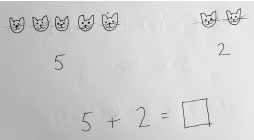(Source: Kindergarten, Module 4, Lesson 16 from EngageNY.org of the New York State Education Department is licensed under CC BY-NC-SA 3.0.) ➔ In Kindergarten, students solve addition and subtraction word problems within 10. (K.OA.A.2) The focus is on solving problems where the result or total is unknown. Grade 3 problems will also focus on finding a result or total, but will require multiplication or division.

 Grade 1 Kea’s boat is 12 centimeters long, and Megan’s boat is 8 centimeters long. How much shorter is Megan’s boat than Kea’s boat?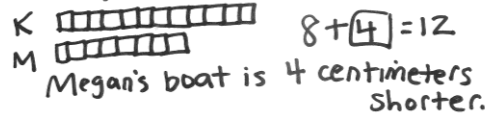(Source: Grade 1, Module 3, Lesson 9 from EngageNY.org of the New York State Education Department is licensed under CC BY-NC-SA 3.0.) ➔ In Grade 1, students solve addition and subtraction word problems within 20. (1.OA.A.1) The focus is on solving compare problems and problems where the change is unknown.

 Grade 2 A pencil costs 59 cents, and a sticker costs 20 cents less. How much do a pencil and a sticker cost together? Solution: The pencil costs 59 cents, and the sticker costs 20 cents less than that: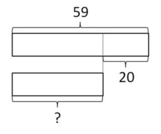So the sticker costs 59-20 = 39 cents.The cost of the two together: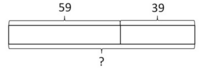is 59 + 39 = 98 cents. (Source: “A Pencil and a Sticker” by Illustrative Mathematics is licensed under CC BY 4.0.) ➔ In Grade 2, students solve one- and two-step addition and subtraction word problems within 100. (2.OA.A.1) The focus is on solving more challenging compare problems and problems where the start is unknown. In this task, students have to find the smaller unknown (e.g., by subtracting 20 from 59) and then find the total. Being able to determine which contexts require which operations becomes especially important as students engage with two-step problems involving all four operations.

Beyond work with addition and subtraction in K-2, a key concept students learn in Grade 1 is the meaning of the equal sign.1.OA.C.7 From there, the foundation for multiplication and division is laid in Grade 2 in students’ work with arrays, partitioning rectangles, and skip-counting. Let’s take a closer look at these three areas and see what they’re all about!

### Grade 2: Work with arrays

Students begin to work with equal groups and arrays and use repeated addition to find the total. (2.OA.C.4) In the following lesson from EnageNY, students use arrays and repeated addition. Students label each row with the size of the group (In Grade 3, students label the rows using a count-by/skip-counting.). Then they use repeated addition to find the total.

#### Grade 2, Module 6, Lesson 6: Concept Development

Distribute materials to students, and instruct them to create the arrays directly on their personal white boards. This way, they can count each row of beans and write the total at the end of the row. Then, they can write the repeated addition equation directly underneath the array.

T: (Show an array of 4 rows of 5 beans with a small space between each bean.)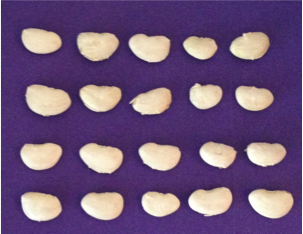T: How many rows do you see?

S: 4 rows.

T: Are my rows equal?

S: Yes!

T: For right now, let’s call a row a group. How many equal groups are there?

S: 4 equal groups.

T: How many beans are in each group?

S: 5 beans.

T: I am going to pull this array apart so we can clearly see our 4 rows. (Using the ruler, separate the rows so there is space between each row as pictured.)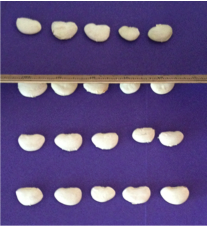T: There are 5 beans in the first row. With your marker, write 5 to the right of the row. (Write 5 to the right of the row.)

T: There are 5 in the second row. (Write 5 to the right of the row as students do the same.) 5 + 5 is…?

S: 10.

T: Add 5 more for the third row. (Write another 5 as students do the same.) 10 + 5 is…?

S: 15.

T: Add 5 more for the last row. (Write another 5 as students do the same.) 15 + 5 is…?

S: 20.T: Look at all these fives! (Point to the 4 fives along the right of the bean array.) What repeated addition equation can we write underneath to show the total number of beans?

S: 5 + 5 + 5 + 5 = 20.

T: Yes! And how many addends do you see?

T: So, there are 4 fives, and 5 + 5 + 5 + 5 equals 20.

HideShow

Later in the same lesson, students label the total in each column and use repeated addition of the columns and observe that the total is the same: 5 + 5 + 5 + 5 = 20 and 4 + 4 + 4 + 4 + 4 = 20. Thus 4 groups of five or 4 fives is the same as 5 groups of 4 or 5 fours.

Similarly, partitioning rectangles is also part of developing a foundation for multiplication. Students partition rectangles into rows and columns of the same-size squares and count to find the total. (2.G.A.2) In the task below, students partition rectangles and use repeated addition of equal addends to find the total number of squares.

#### Partitioning a Rectangle into Unit Squares

Materials

• Copies of a rectangle with edges marked (one for each student/ group, see attached blackline master)
• A straight edge tool

Actions

The teacher should guide students through these actions, as the text in this task is too complex for some second graders.

1. Draw a grid on the rectangle by connecting each mark to the one directly across from it on the opposite edge.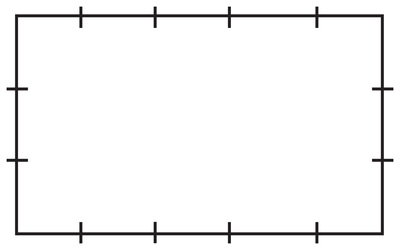1. The grid separates the rectangle into many little squares. How many squares are there?
2. There are five little squares in each row. Count by fives to find how many squares there are in the entire rectangle.
3. What other methods can you think of to quickly count how many squares there are in the entire rectangle?
4. Write a number in each little square to count them and show that your answers are correct.
5. One number sentence which shows the total number of squares is 3 + 3 + 3 + 3 + 3 = 15. Write another number sentence which shows the total number of squares.

HideShow

Students also explore even and odd numbers and write an equation to express an even number as the sum of two equal addends. (2.OA.C.3) In the exploration of even numbers, students do a lot of counting by twos and doubling using arrays and pairs of objects. Counting by twos and doubling supports students with their multiplication facts of twos.

Lastly, students prepare for multiplication and division through skip-counting, which helps support Level 2 methods for multiplying and dividing. Skip-counting actually begins in Kindergarten, with counting by tens to 100. (K.CC.A.1) In Grade 2, students skip-count by 5s, 10s, and 100s (and by 2s when pairing even numbers). (2.NBT.A.2) Daily skip-counting with students helps students move from Level 1 strategies to Level 2 strategies (from count all to count on by). This activity shows one way to practice skip-counting as part of a daily routine:

#### EngageNY Grade 2, Module 3, Lesson 1: Fluency Practice

Skip-Count Up and Down by Fives on the Clock (11 minutes)

Materials: (T) A “clock” made from a 24-inch ribbon marked off at every 2 inchesT: (Display the ribbon as a horizontal number line—example pictured above.) Count by fives as I touch each mark on the ribbon.

S: (Starting with 0, count by fives to 60.)

T: (Make the ribbon into a circle resembling a clock.) Now I’ve shaped my ribbon to look like a …

S: Circle! Clock!

T: Let’s call it a clock. Again, count by fives as I touch each mark on the clock.

S: (Starting with 0, skip-count by fives to 60.)

T: This time, the direction my finger moves on the clock will show you whether to count up or down. (While explaining, demonstrate sliding a finger forward and backward around the clock.)

T: As I slide to the marks, you count them by fives.

HideShow

### Suggestions for students who are below grade level

If students come to Grade 3 without a solid grasp of the ideas named above (or haven’t encountered them at all), what can you do? It’s not practical (or even desirable) to reteach everything students should have learned in Grades K-2; there’s plenty of new material in Grade 3, so the focus needs to be on grade-level standards. At the same time, there are strategic ways of wrapping up “unfinished learning” from prior grades. Here are a few suggestions for adapting your instruction to bridge the gaps with respect to preparedness for multiplication and division with whole numbers.

• We’ve talked about the way that Grade 3 emphasizes Level 3 strategies for multiplication and division; however, some of your students may still be employing “counting all” to solve multiplication and division problems (i.e., Level 1). In this case, additional instruction and practice with skip-counting will help bring students along to Level 2 methods. Consider a daily fluency warm-up that gives students time to learn and practice this skill, such as the warm-up activity in this lesson. Once students are able to solve multiplication and division problems by skip-counting, they will be better positioned to learn the Level 3 methods associated with Grade 3.
• If a significant number of your students lack the conceptual background provided by visual representations of equal groups, work with arrays and partitioning rectangles may offer necessary scaffolding. Planning a few introductory lessons that ask students to analyze and write repeated addition equations from arrays or tiled rectangles to begin a unit on multiplication and division can support students with these prerequisites. This module provides lessons that introduce students to arrays (Topic B) and partitioning rectangles (Topic C).

## Beyond Grade 3: What’s next with multiplication and division?

It is helpful to understand how students will build on their understanding of multiplication and division in later grades. Understanding the progression of this content can help to solidify the focus in third grade. It helps us to define the limits of our instruction in Grade 3 and helps us see why we focus so intently on a small number of concepts. At the same time, we realize that we want to set our students up for future success, and knowing the next steps in their journey can help us focus our lessons on the knowledge and skills that matter most.

### Grades 3-5: A progression of meaning for multiplication and division

The progression for multiplication and division in Grades 3-5 is very purposeful. In Grade 3, students develop an understanding of the meaning of multiplication and division of whole numbers through activities and problems involving equal-sized groups, arrays, and area models. Students build relationships between multiplication and division over time such that division is understood as reversing the action of multiplication and as finding an unknown factor. Students come to understand the properties of multiplication and use these properties to solve problems.24 Progressions for the Common Core State Standards in Mathematics (draft): K, Counting and Cardinality; K–5, Operations and Algebraic Thinking, pp. 2-3. Progressions for the Common Core State Standards in Mathematics (draft): K, Counting and Cardinality; K–5, Operations and Algebraic Thinking, pp. 2-3. Progressions for the Common Core State Standards in Mathematics (draft): K, Counting and Cardinality; K–5, Operations and Algebraic Thinking, pp. 2-3.

In fourth grade, students build on their understanding of multiplication and division as equal groups and array/area problems. Students learn to interpret multiplication equations as comparisons and solve multiplication and division word problems involving multiplicative comparisons. (4.OA.A.1, 4.OA.A.2) Students learn to distinguish multiplicative comparison from additive comparisons (the idea that one quantity is simply so many more than another). The differences between these concepts are shown below:

 Multiplicative comparison “My friend has 2 apples and I have 10 apples, so I have 5 times as many apples as she does.” 2 × ___ = 10 Additive comparison “My friend has 2 apples and I have 10 apples, so I have 8 more apples than she does.” 2 + ___ = 10

In fifth grade students extend their understanding of multiplicative comparisons to interpret multiplication as scaling, including the use of fractions.5.NFB.B.3 Taking a look at tasks from each grade helps illustrate the progression of meaning for multiplication:

 Grade 3 Liam bought 5 bunches of bananas. Each bunch has exactly 5 bananas. How many bananas does Liam have? Mrs. Oro needs 90 corn seeds. The Garden Center sells corn seeds in packs of 10 seeds each. Write a division equation showing how many packs of seeds Mrs. Oro should buy. (Source: “Introducing Multiplication and Division Unit Plan” by Student Achievement Partners is licensed under CC 0 1.0.) ➔ With multiplication and division problems like these, students use the ideas of multiplication as equal groups and division as an unknown factor. These are the foundation for all future work involving multiplication and division.

 Grade 4 The Turner family uses 548 liters of water per day. The Hill family uses 3 times as much water per day. How much water does the Hill family use per day? How much water will they use per week? (Source: Grade 4, Module 3, Lesson 12 (teacher version) from EngageNY.org of the New York State Education Department is licensed under CC BY-NC-SA 3.0.) ➔ With multiplicative comparison problems like this one, students advance beyond thinking of equal groups to more sophisticated thinking about comparison.

### Grades 3-5: Toward fluency with the standard algorithm for multiplying whole numbers

Knowing the products of two one-digit numbers from memory in Grade 3 is important for continued work with multiplication in Grades 4 and 5. In Grade 4, students multiply a whole number of up to four digits by a one-digit whole number, and multiply two two-digit numbers, using strategies based on place value and the properties of operations. (4.NBT.B.5) Additionally, students find whole-number quotients and remainders with up to four-digit dividends and one-digit divisors, using strategies based on place value, the properties of operations, and/or the relationship between multiplication and division. (4.NBT.B.6 )It’s important to point out that in Grade 4 multiplying and dividing with larger numbers is still supported by using strategies based on place value, properties of operations, the relationship between multiplication and division, and through arrays and area models. In other words, the same concepts and strategies developed in Grade 3. The example below illustrates using the distributive property, in the context of an area model, to solve a two-step problem involving equal groups (i.e., 9 x 2,650 = 9 x (2,000 + 600 + 50) = (9 x 2,000) + (9 x 600) + (9 x 50).

#### Grade 4, Module 3, Lesson 11: Problem Set

1. A restaurant sells 1,725 pounds of spaghetti and 925 pounds of linguini every month. After 9 months, how many pounds of pasta does the restaurant sell?Finally, students are expected to fluently use the standard algorithm for multiplication by the end of Grade 5 (5.NBT.B.5 )and by end of Grade 6 for division. (6.NS.B.2 )Students use visuals like area models to provide meaning for algorithms, which follow from understanding of the properties and strategies in Grades 3 and 4. The example below shows how a student might support multi-digit multiplication using an area model and connecting that area model to the standard algorithm.

#### EngageNY Grade 5, Module 2, Lesson 6: Concept Development

Problems 2 – 3

814 × 39

624 × 82

T: (Write 814 × 39 on the board.) Partner A, use the standard algorithm to solve. Partner B, draw an area model to solve.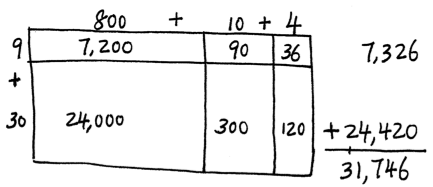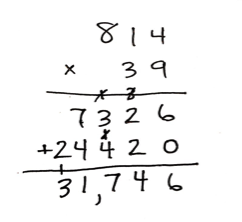S: (Draw and solve.)

HideShow

Examples like these are an important part of the work in Grade 5; students become comfortable with the standard algorithm by connecting it to prior work with visual models and other strategies.

Without fluency with one-digit products and associated quotients, work with later multiplication and division becomes compromised.

Student Achievement Partners: Focus in Grade 3

EngageNY: Grade 3 Module 1 Materials

# Endnotes

 The Common Core State Standards for Mathematics (CCSSM) are organized into major, additional and supporting clusters in the Focus by Grade Level documents from Student Achievement Partners.

 To understand what is considered major, supporting and additional work in Grade 2, or other grades, see the Focus by Grade Level documents from Student Achievement Partners.

 In Grades K-2, students focus extensively on representing and solving word problems involving addition and subtraction of whole numbers within 100. Table 1 from CCSSM describes the different problem-solving situations students are expected to master by the end of Grade 2. Students are expected to continue to engage with these problem-solving situations in Grades 3-5; however, students use larger numbers, numbers other than whole numbers, and they combine the use of several operations to solve multi-step word problems. It is important to become familiar with the problem-solving situations described in Table 1. Additionally, students who have difficulty understanding problem-solving involving multiplication and division may lack sufficient understanding of problem-solving involving addition and subtraction.

 Adapted from CCSSM Table 2

 Footnote 3 from CCSSM Table 2: The first examples in each cell are examples of discrete things. These are easier for students and should be given before the measurement examples.

 Progressions for the Common Core State Standards in Mathematics (draft): K, Counting and Cardinality; K–5, Operations and Algebraic Thinking, p. 24.

 Ibid. p. 25.

 The Level 1 method for addition and subtraction is counting all, Level 2 is counting on, and Level 3 is making an easier problem by decomposing and composing numbers. See a discussion on these levels in the Grade 1 Guide.

 Progressions for the Common Core State Standards in Mathematics (draft): K, Counting and Cardinality; K–5, Operations and Algebraic Thinking, pp. 25-26.

 Progressions for the Common Core State Standards in Mathematics (draft): K, Counting and Cardinality; K–5, Operations and Algebraic Thinking, p. 25.

 Table 3 of the CCSSM describes the properties of operations

 Progressions for the Common Core State Standards in Mathematics (draft): K, Counting and Cardinality; K–5, Operations and Algebraic Thinking, p. 27.

 To see a detailed exploration of patterns with multiplying by 9, review lessons 12-14 in Module 3 of the EngageNY curriculum.

 Progressions for the Common Core State Standards in Mathematics (draft): K, Counting and Cardinality; K–5, Operations and Algebraic Thinking, p. 26.

 Mnemonic devices such as PEMDAS offer students an incomplete picture of the concepts at hand; applying these rules without deeper understanding often leads to incorrect mathematics. For example, the expression 6 – 2 + 3, according to the PEMDAS rule, is computed with addition first: 6 – (2 +3) = 6 – 5 = 1. However, the correct computation involves addition and subtraction as they appear from left to right: 6 – 2 + 3 = 4 + 3 = 7.

 Progressions for the Common Core State Standards in Mathematics (draft): K, Counting and Cardinality; K–5, Operations and Algebraic Thinking, p. 28.

 The idea that standards relate strongly to one another is known as coherence, and is a distinctive feature of the Common Core State Standards for Mathematics. If you’re interested in exploring more of the connections between standards, you might want to check out the Student Achievement Partners Coherence Map, which illustrates them visually.

 The Common Core State Standards for Mathematics (CCSSM) are organized into major, additional, and supporting clusters in the Focus by Grade Level documents from Student Achievement Partners.

 The word problem situations for addition and subtraction and when they are introduced can be found on page 9 of The Progressions document: Progressions for the Common Core State Standards in Mathematics (draft): K, Counting and Cardinality; K–5, Operations and Algebraic Thinking, p. 9. The different problem situations are also relevant in Grades 3-5 when students engage in addition and subtraction situations with larger whole numbers, decimals, and fractions.

 Progressions for the Common Core State Standards in Mathematics (draft): K, Counting and Cardinality; K–5, Operations and Algebraic Thinking, p. 18-19.

 Progressions for the Common Core State Standards in Mathematics (draft): K, Counting and Cardinality; K–5, Operations and Algebraic Thinking, p. 19.

 Progressions for the Common Core State Standards in Mathematics (draft): K, Counting and Cardinality; K–5, Operations and Algebraic Thinking, pp. 2-3.

# FAQs

## 1. What is a Content Guide?

Our goal in creating the Content Guides has been to provide busy teachers with a practical and easy-to-read resource on what the grade-level math standards are saying, along with examples of instructional materials that support conceptual understanding, problem-solving, and procedural skill and fluency for students.

It’s important to note that content guides are not meant to serve as a curriculum (or any kind of student-facing document), a guide or source material for test-preparation activities, or any kind of teacher evaluation tool.

## 2. What’s in a Content Guide?

Each Content Guide is focused on a specific group of standards. Most Content Guides follow the same three-part structure:

• Part 1 makes clear the student skills and understandings described by this group of standards. This section illustrates the standards using multiple student tasks from freely available online sources. Teachers can use or adapt these tasks for their students.
• Part 2 explains how this group of standards is connected to other standards in the same grade. We highlight how these connections have implications for planning and teaching, and how this within-grade coherence can increase access for students. Part 2 also includes multiple student tasks from freely available online sources.

• Part 3 traces selected progressions of learning leading to grade-level content discussed in the specific Content Guide. This discussion segues into a series of concrete and practical suggestions for how teachers can leverage the progressions to teach students who may not be prepared for grade-level mathematics. Finally, Part 3 traces the progression to content in higher grades.

## 3. How can I use the Content Guides?

Teachers who have read our Content Guides say they see benefits for all educators. Here are some suggestions for how different educators might use them.

Teachers can use the Mathematics Content Guides to:

• Increase or refresh their knowledge of the standards and the expectations for what students should know by the end of the year.
• Adapt lessons and units using appropriate pre-requisites to support students who are behind grade-level.
• Gain access to the best available OER for math to use for introducing and/or reinforcing concepts
• Ensure their curriculum and/or units:
• Focus on the major work of the grade and the appropriate depth of each standard.
• Target the appropriate aspects of rigor—procedural skill and fluency, modeling and application, and conceptual understanding described by the standards.
• Help students make coherent connections within and across grades.
• Create or revise their lessons and questioning to focus on important concepts in the standards.

Instructional coaches and school leaders can use the Mathematics Content Guides to:

• Refresh or increase their knowledge of the standards and the expectations for what students should know by the end of the year.
• Develop and communicate consistent expectations for lesson planning and instruction aligned to the standards.
• Provide a reference when planning and/or discussing instruction with teachers.
• Gain insight into what instruction and student work should look like in order to meet the demands of the standards.
• Develop and design content and standards-driven professional development sessions/workshops.
• Foster content rich, standards-based discussions among staff and build staff knowledge.
• Develop and/or revise school improvement plans in order to support and incorporate content and practice-based teaching and learning.

## 4. Why the Content Guides?

The transition to higher standards has led teachers all over the country to make significant changes in their planning and instruction, but only one-third of teachers feel they are prepared to help their students pass the more rigorous standards-aligned assessments (Kane et.al., 2016). This is to be expected because the new high standards are a significant departure from prior standards. The standards require a deeper level of understanding of the math content they teach; a different progression of what students need to learn by which grade; as well as different pedagogy that emphasizes student conceptual understanding, problem solving and procedural fluency in equal intensity.

The support for teachers to bring high standards to their classrooms, however, has lagged behind. Research shows that teacher training in the U.S. is currently insufficient in preparing teachers to teach the demanding new standards (Center for Research in Mathematics and Science Education, 2010). And though some resources exist that “unpack” the standards, few, if any, explain and illustrate the standards. “Unpacking” the standards one by one can also result in a disjointed presentation that neglects the structure and coherence of the standards. In creating the Content Guides, we aimed to provide busy teachers with a practical, easy-to-read resource on their grade-specific standards and how to help all students learn them. There is ample empirical evidence that when teachers have both strong knowledge of the math content that they teach, and the pedagogical knowledge to help students master that content knowledge, their students learn more (Baumert et. al., 2010; Hill, Rowan and Ball, 2005; Rockoff et. al., 2008). With the Content Guides in hand, we hope that teachers will find more success in helping their students make progress toward college- and career-readiness.

## 5. What is the relationship between the Content Guides and the Progressions?

The Progressions documents describe the grade-to-grade development of understanding of mathematics. These were informed by research on children’s cognitive development as well as the logical structure of mathematics. The Progressions explain why standards are sequenced the way they are. The Content Guides often highlight key ideas from the Progressions, but do not add new standards or change the expectations of what students should know and be able to do; they aim to explain and illustrate a group of standards at a time using freely available online sources. While the OER tasks and lessons in the Content Guides are one way to meet the grade-level standards, they are not the only means for doing so.

## 6. How were the resources selected?

We selected sample tasks and lessons from freely available online sources such as EngageNY, Illustrative Mathematics and Student Achievement Partners to illustrate the Standards. These sources are chosen because they are fully aligned to the new high standards based on national review of K-12 curricula or are created by organizations led by the writers of the new high standards. In addition, because they are open educational resources (OER), they are freely accessible for all uses. All UnboundEd materials are also OER, as part of our commitment to make high-quality, highly aligned content available to all educators.